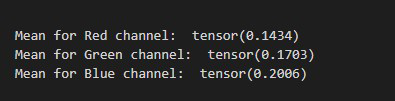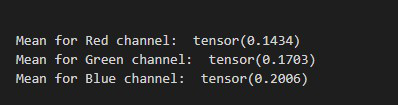Open in App
Not now

# How to Find Mean Across the Image Channels in PyTorch?

• Last Updated : 03 Jun, 2022

In this article, we are going to see how to find mean across the image channels in PyTorch. We have to compute the mean of an image across the channels Red, Green, and, Blue. we can find the mean across the image channel by using torch.mean() method.

## torch.mean() method

torch.mean() method is used to find the mean of all elements in the input tensor but this method only accepts input as a tensor only so first we have to convert our image to a PyTorch tensor. After converting we use this PyTorch tensor as the input tensor. The below syntax is used to find mean across the image channels

Syntax: torch.mean(input, dim)

Parameter:

• input (Tensor): This is our input tensor.
• dim (int or tuple of python:ints): the dim is used for dimensions. we set dim = [1,2] to find mean across the image channels Red, Green, and Blue.

Return: This method returns the mean for all the elements present in the input tensor.

The below image is used for Example:Example 1: In the below example, we use PIL to read images from the computer and then we find mean across the image channels in PyTorch.

## Python

 `# import required libraries` `import` `torch` `from` `PIL ``import` `Image` `import` `torchvision.transforms as transforms`   `# Read input image` `img ``=` `Image.``open``(``'img.png'``)`   `# create a transform` `transform ``=` `transforms.ToTensor()`   `# convert the image to PyTorch Tensor` `imgTensor ``=` `transform(img)`   `# Compute the mean of Image across the ` `# channels RGB` `r, g, b ``=` `torch.mean(imgTensor, dim``=``[``1``, ``2``])`   `# Display Result` `print``(``"Mean for Red channel: "``, r)` `print``(``"Mean for Green channel: "``, g)` `print``(``"Mean for Blue channel: "``, b)`

Output:Example 2: In the below example, we use OpenCV to read images from the computer and then we find mean across the image channels in PyTorch.

## Python

 `# import required libraries` `import` `torch` `import` `cv2` `import` `torchvision.transforms as transforms`   `# Read input image using OpenCV` `img ``=` `cv2.imread(``'img.png'``)` `img ``=` `cv2.cvtColor(img, cv2.COLOR_BGR2RGB)`   `# create a transform` `transform ``=` `transforms.ToTensor()`   `# convert the image to PyTorch Tensor` `imgTensor ``=` `transform(img)`   `# Compute the mean of Image across the ` `# channels RGB` `r, g, b ``=` `torch.mean(imgTensor, dim``=``[``1``, ``2``])`   `# Display Result` `print``(``"\n\nMean for Red channel: "``, r)` `print``(``"Mean for Green channel: "``, g)` `print``(``"Mean for Blue channel: "``, b)`

Output:My Personal Notes arrow_drop_up
Related Articles